International
Tables for
Crystallography
Volume A
Space-group symmetry
Edited by M. I. Aroyo

International Tables for Crystallography (2016). Vol. A, ch. 3.4, pp. 796-798

## Section 3.4.2. The concept of characteristic and non-characteristic orbits, comparison with the lattice-complex concept

W. Fischera and E. Kocha*

aInstitut für Mineralogie, Petrologie und Kristallographie, Philipps-Universität, D-35032 Marburg, Germany
Correspondence e-mail:  kochelke@mailer.uni-marburg.de

### 3.4.2. The concept of characteristic and non-characteristic orbits, comparison with the lattice-complex concept

| top | pdf |

#### 3.4.2.1. Definitions

| top | pdf |

The generating space group of any crystallographic orbit may be compared with the eigensymmetry of its point configuration. If both groups coincide, the orbit is called a characteristic crystallographic orbit, otherwise it is named a non-characteristic crystallographic orbit (Wondratschek, 1976; Engel et al., 1984; see also Section 1.1.7). If the eigensymmetry group contains additional translations in comparison with those of the generating space group, the term extraordinary orbit is used (cf. also Matsumoto & Wondratschek, 1979). Each class of configuration-equivalent orbits contains exactly one characteristic crystallographic orbit.

The set of all point configurations incan be divided into 402 equivalence classes by means of their eigensymmetry: two point configurations belong to the same symmetry type of point configuration if and only if their characteristic crystallographic orbits belong to the same type of Wyckoff set.

As each crystallographic orbit is uniquely related to a certain point configuration, each equivalence relation­ship on the set of all point configurations also implies an equivalence relationship on the set of all crystallo­graphic orbits: two crystallographic orbits are assigned to the same orbit type (cf. also Engel et al., 1984) if and only if the corresponding point configurations belong to the same symmetry type.

In contrast to lattice complexes, neither symmetry types of point configuration nor orbit types can be used to define equivalence relations on Wyckoff positions, Wyckoff sets or types of Wyckoff set. Two crystallographic orbits coming from the same Wyckoff position belong to different orbit types, if – owing to special coordinate values – they differ in the eigensymmetry of their point configurations. Furthermore, two crystallographic orbits with the same coordinate description, but stemming from different space groups of the same type, may belong to different orbit types because of a specialization of the metrical parameters.

#### Example

The eigensymmetry of orbits from Wyckoff position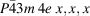with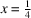or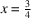is enhanced to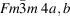and hence they belong to a different orbit type to those with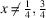.

#### Example

In general, an orbit belonging to the type of Wyckoff set I4/m 2a, b corresponds to a point configuration with eigensymmetry I4/mmm 2a, b. If, however, the space group I4/m has specialized metrical parameters, e.g. c/a = 1 or c/a = 21/2, then the eigensymmetry of the point configuration is enhanced to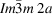or, respectively.

#### 3.4.2.2. Comparison of the concepts of lattice complexes and orbit types

| top | pdf |

It is the common intention of the lattice-complex and the orbit-type concepts to subdivide the point configurations and crystallographic orbits ininto subsets with certain common properties. With only a few exceptions, the two concepts result in different subsets. As similar but not identical symmetry considerations are used, each lattice complex is uniquely related to a certain symmetry type of point configuration and to a certain orbit type, and vice versa. Therefore, the two concepts result in the same number of subsets: there exist 402 lattice complexes and 402 symmetry types of point configuration and orbit types. The differences between the subsets are caused by the different properties of the point configurations and crystallographic orbits used for the classifications (cf. also Koch & Fischer, 1985).

The concept of orbit types is entirely based on the eigensymmetry of the particular point configurations: a crystallographic orbit is regarded as an isolated entity, i.e. detached from its Wyckoff position and its type of Wyckoff set. On the contrary, lattice complexes result from a hierarchy of classifications of crystallographic orbits into Wyckoff positions, Wyckoff sets, types of Wyckoff set and classes of configuration-equivalent types of Wyckoff set, i.e. a crystallographic orbit is always considered as being embedded in its type of Wyckoff set, and the eigensymmetry of a particular point configuration is disregarded. The differences between the two concepts become clear if limiting complexes are considered.

Forty-nine lattice complexes without any limiting complex exist (cf. Table 3.4.2.1). They coincide completely with the corresponding symmetry types of point configurations. As can be extracted from the tables by Engel et al. (1984) there exist 15 additional lattice complexes without limiting complexes due to specialized coordinates. For fundamental reasons, no cubic or hexagonal complexes allow any metrical specialization.

#### Example

The lattice complex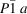of all triclinic point lattices includes as limiting complexes the 13 other lattice complexes that refer to Bravais lattices. Hence the crystallographic orbits ofbelong to 14 different orbit types.

 Table 3.4.2.1| top | pdf | Reference symbols of the 28 lattice complexes with f ≥ 1 degrees of freedom without any limiting complex
Lattice complexfLattice complexf
P4/mmm l 1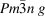1
P42/mmc j 1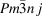1
I4/mmm i 1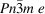1
P6222 g 1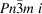1
P6/mmm l 1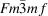1
P6/mmm p 2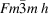1
P4232 k 1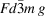2
I432 i 1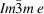1
I4132 h 1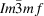1
I4132 i 3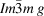1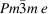1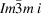1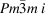1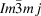2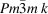2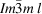3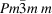2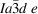1

#### Example

The lattice complex Fddd a of all orthorhombic diamond patterns includes as limiting complexes those of the tetragonal and the cubic diamond patterns I41/amd a and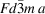, respectively. The orbits of Fddd a with specialized metric, therefore, belong to the orbit types I41/amd a or.

353 lattice complexes comprise at least one limiting complex. Each of them includes additional point configurations in comparison to the corresponding symmetry type of point configuration (and orbit type), namely those belonging to the limiting complex.

#### Example

Lattice complex24gcomprises for y = z the limiting complex24h, and for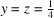the limiting complex3c. The corresponding orbits with y = z anddo not belong to orbit type24g.

#### Example

P4/mmm 8rcomprises for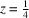the limiting complex P4/mmm 4j, forthe limiting complex P4/mmm 2g, for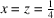the limiting complex P4/mmm 1a, for a = c and x = z the limiting complex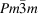8g, and for a = c andthe limiting complexla. Again, none of the corresponding orbits belong to orbit type P4/mmm 8r.

The comparison of an orbit type with its corresponding lattice complex is more intricate. Again, the concept of limiting complexes and comprehensive complexes elucidates the interrelation.

Let A be a lattice complex with a limiting complex B and a comprehensive complex C. The respective orbit types will also be designated A, B and C (e.g. A =24h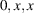; B =3c, d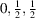,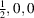; C =24g). Then a crystallographic orbit from a Wyckoff position of lattice complex A belongs to orbit type A only if it does not correspond to a point configuration of the limiting complex B (i.e. only the crystallographic orbits of24h with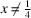belong to orbit type24h). The crystallographic orbits of lattice complex A, however, that do correspond to the limiting complex B belong to orbit type B (i.e. all crystallographic orbits from24h withbelong to orbit type3c, d). On the contrary, those orbits that refer to lattice complex C and that happen to correspond to the limiting complex A of C belong to orbit type A instead of orbit type C. All crystallographic orbits of24gwith y = z orcreate point configurations of lattice complex24g but belong to orbit type24h or3c, d, respectively.

For the comparison of lattice complexes and orbit types the concept of non-characteristic orbits is less helpful than the concept of limiting complexes. In terms of lattice complexes, there exist two basically different reasons for a crystallographic orbit to be non-characteristic:

 (1) The crystallographic orbit under consideration belongs to a non-characteristic type of Wyckoff set of a lattice complex. Then this orbit, together with all other orbits from its type of Wyckoff set, is non-characteristic. A characteristic crystallographic orbit necessarily stems from a characteristic Wyckoff set of a lattice complex. (2) The crystallographic orbit under consideration stands out with respect to the eigensymmetry of its point configuration compared with the other orbits out of its type of Wyckoff sets, i.e. it corresponds to a limiting complex. Then this orbit, together with all other orbits referring to that limiting complex, is non-characteristic.

As a consequence, three kinds of non-characteristic orbits may be distinguished:

 (1) those that belong to a non-characteristic Wyckoff set, but do not correspond to a limiting complex, e.g. all orbits from6e to h; (2) those that belong to a characteristic Wyckoff set, but correspond to a limiting complex, e.g.8gwithor P4/mmm 1a, b with a = c; (3) those that belong to a non-characteristic Wyckoff set and, in addition, correspond to a limiting complex, e.g.8iwith.

As these considerations illustrate, limiting complexes and non-characteristic orbits do not coincide and a statement by Engel (1983) proposing this correspondence, therefore, is not correct.

The concept of lattice complexes and limiting complexes on the one hand and of orbit types and non-characteristic orbits on the other hand are complementary in a certain sense: it is possible to derive all orbit types and all non-characteristic orbits from the complete knowledge of lattice complexes and limiting complexes and vice versa.

Engel et al. (1984) enumerated for all space-group types those non-characteristic orbits that refer to special coordinates, but they excluded all further ones that are based on specialized metrical parameters of the generating space groups or on the simultaneous specialization of metrical and coordinate parameters. A computer program which enables the determination of non-characteristic orbits is now available (NONCHAR on the Bilbao Crystallographic Server at http://www.cryst.ehu.es ). Lawrenson & Wondratschek (1976) listed the extraordinary orbits of the plane groups, and Matsumoto & Wondratschek (1987) listed the non-characteristic orbits of the plane groups.

The special, but not exceptional, case in which a non-characteristic orbit is produced only if both the coordinates and metric are specialized deserves extra concern. The crystallographic orbits from6fwith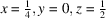or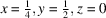and with the rhombohedral angle α = 90° may be used as an example. The eigensymmetry of the corresponding point configurations is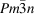6c, d (corresponding to the position of the Cr atoms in the crystal structure of Cr3Si). Accordingly, the lattice complexf comprises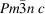as limiting complex.shows special integral reflection conditions (hkl: h + k + l = 2n or h = 2n + 1, k = 4n, l = 4n + 2; h, k, l permutable), which of course hold for all orbits of that type, i.e. also for the special orbits fromf described above. As geometrical structure factors are independent of metrical parameters, these reflection conditions are even valid for crystallographic orbits fromf with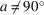if the coordinates are restricted to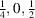or to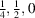.

In general, the following statement holds: if a lattice complex causes special reflection conditions then exactly these conditions are also valid for any crystallographic orbit that refers to a comprehensive complex of that lattice complex if, in addition, this crystallographic orbit may be described by the same coordinate triplets as an orbit of the lattice complex under consideration.

### References

Engel, P. (1983). Zur Theorie der kristallographischen Orbits. Z. Kristallogr. 163, 243–249.
Engel, P., Matsumoto, T., Steinmann, G. & Wondratschek, H. (1984). The non-characteristic orbits of the space groups. Z. Kristallogr. Supplement issue No. 1.
Koch, E. & Fischer, W. (1985). Lattice complexes and limiting complexes versus orbit types and non-characteristic orbits: a comparative discussion. Acta Cryst. A41, 421–426.
Lawrenson, J. E. & Wondratschek, H. (1976). The extraordinary orbits of the 17 plane groups. Z. Kristallogr. 143, 471–484.
Matsumoto, T. & Wondratschek, H. (1979). Possible superlattices of extraordinary orbits in 3-dimensional space. Z. Kristallogr. 150, 181–198.
Matsumoto, T. & Wondratschek, H. (1987). The non-characteristic G-orbits of the plane groups G. Z. Kristallogr. 179, 7–30.
Wondratschek, H. (1976). Extraordinary orbits of the space groups. Theoretical considerations. Z. Kristallogr. 143, 460–470.